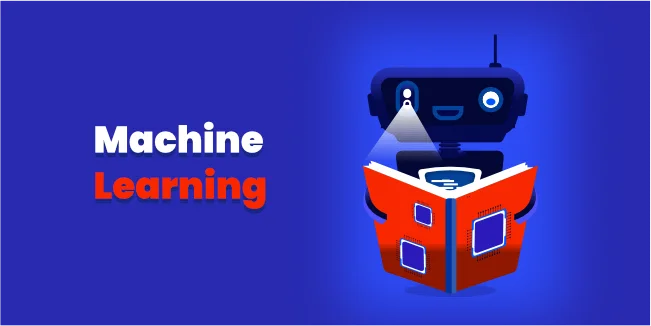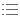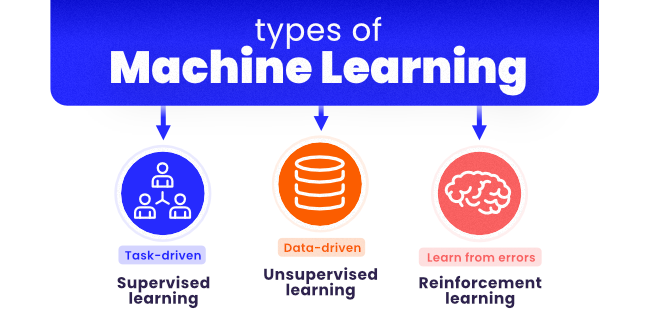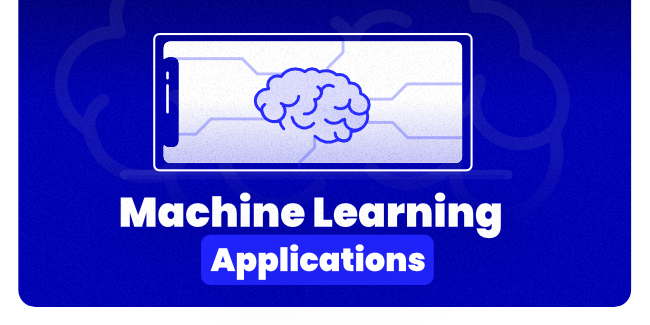2022-01-11

ai
ml
machinelearning

# Machine Learning explainedContents

Machine Learning is a process of teaching computers to learn from data without being explicitly programmed. A subset of artificial intelligence enables computers to learn how to do things independently by analyzing examples and making predictions. Machine Learning algorithms can make predictions about future events, recognize patterns in data, and optimize business processes.

There are many different Machine Learning algorithms, but they all rely on the same basic principle: given a large enough sample of data, a computer can learn to identify patterns and make predictions. The computer is not told what to look for ahead of time; it simply looks at the data and tries to find relationships between input and output variables. Once it finds a pattern, it can use it to make predictions about future data sets.

One of the most common applications of machine learning is predictive analytics. Predictive analytics uses historical data to predict future outcomes. For example, a business might want to use predictive analytics to predict how many widgets it will sell next week. To do this, the business would collect data on past sales of widgets and use a machine-learning algorithm to analyze that data. The algorithm would identify patterns in the data and use them to make predictions about future sales.

Machine learning can also be used to improve business processes. For example, a company might use machine learning algorithms to optimize its supply chain. The algorithms would identify patterns in the data related to inventory levels, shipping times, and demand. These patterns would then be used to inform decisions about how much product to produce, when to have it shipped, and how much demand to expect.

In the business world, the term "machine learning" is often being used as a synonym for predictive analytics or artificial intelligence (AI). While there is a connection between machine learning and these concepts, they are not the same thing. In fact, machine learning can be thought of as a type of AI where instead of programming an algorithm with rules and logic based on known patterns in the data being analyzed, one trains an algorithm by showing it example data sets that demonstrate those patterns.

This training process works by giving the algorithm many examples of inputs and their desired outputs until it learns which inputs produce which outputs. The algorithm does this by finding patterns in the data that allow it to make its own estimations on new inputs, allowing for predictions to be made without having ever seen those inputs before.

The idea of an algorithm learning about patterns in the data is often confused with AI since machine learning tasks are commonly used for many advanced applications such as image recognition, speech-to-text conversion, and natural language processing. However, although these algorithms can function autonomously after being trained, they actually act more like "filters" than they do than general artificial intelligence.

## How Machine Learning works?

Machine Learning uses predictive models that generate inferences from existing datasets (examples) to draw conclusions or predictions about new data. For a machine-learning algorithm to be effective, it must first be "trained" using a set of known inputs and outputs. The algorithm will then analyze the relationships between these input and output values to identify patterns or trends. Once these patterns have been identified, the algorithm can use them to predict future data points.

### Seven Steps of Machine Learning

• Gathering Data
• Preparing that data
• Choosing a model
• Training
• Evaluation
• Hyperparameter Tuning
• Prediction

### Three major blocks of a Machine Learning

The three major building blocks of a Machine Learning system are the model (we describe Machine Learning models below), the learner's parameters.

• Model is the system that makes predictions
• The parameters are the factors that the model considers to make predictions
• The learner makes the parameters and the model adjustments to align the predictions with the actual results

Let's assume we're using Machine Learning to predict whether a drink is water or fizzy. In this instance, the model must predict if the beverage is water or fizzy. The beverage's color and the carbonation level are the two variables selected.

### Learning from the training set

Take a sample data set of several beverages for which the color and carbonation percentage are specified. Now, we must describe each classification, such as water and fizzy, in terms of the parameter values for each type. The model can use the description to determine if a new drink is water or fizzy. The set of data that goes into making a trained model is known as a training set. The parameters of each drink in the training data, such as 'color' and 'carbonation percentages,' can be represented by 'x' and 'y.' Then (x,y) is used to define the parameters of each drink in the training data.

### Measuring errors

After the model has been trained on known past data, it must be checked for errors and anomalies. We utilize new data to do this work. One of these four outcomes will result:

• True Positive: When the model predicts the condition when it is present
• True Negative: When the model does not predict a condition when it is absent
• False Positive: When the model predicts a condition when it is absent
• False Negative: When the model does not predict a condition when it is present. The sum of FP and FN is the total error in the model

### Manage Noise

We've only considered two variables in our attempts to solve a Machine Learning problem here: color and carbonation percentage. However, to solve a Machine Learning issue, you'll have to consider hundreds of factors and a wide range of learning data.

Noise is any irregularity in the data set that obscures the underlying connection and hampers learning. There are several causes for this noise to emerge: Large training data set; Errors in input data; Data labeling errors; Attributes that are unobservable and may influence the classification but for lack of data aren't considered in the training set. Because of the noise, your hypothesis will have a lot more mistakes.

To maintain the hypothesis as basic as feasible, you may accept a certain amount of noise training error.

### Testing and Generalisation

While an algorithm or hypothesis may fit well in a training set, it may not perform as expected when put to new data. As a result, determining if the method is appropriate for fresh material is critical. Testing it on new data is the best method to determine this. Also, generalization refers to how well the model performs with new data.

The training data may have less error when we fit a hypothesis algorithm for maximum possible simplicity, but the new data might have greater significance. If the hypothesis is too complicated to fit the best match to the training result properly, it won't be able to generalize well. Underfitting and overfitting are terms that describe this. It is known as over-fitting. Under or overfitting, the findings are fed back into training to improve the model.

## Types of Machine Learning### Explanations of the most popular Machine Learning models

Model is the system that makes predictions. Supervised and unsupervised learning models are the two basic types of Machine Learning. A supervised model is further divided into either a regression or classification type if a model is a supervised model. We can also highlight semi-supervised Machine Learning.

### Supervised Machine Learning

A supervised learning model, also known as supervised pattern recognition or supervised learning algorithms, is a Machine Learning model, which teaches a computer how to perform a task by providing it with example input-output pairs.

### Regression

In regression models, the output is continuous. The following is a selection of the most frequent types of regression models.

The most popular regression algorithms are:

• Ordinary Least Squares Regression (OLSR)
• Linear Regression
• Logistic Regression
• Stepwise Regression
• Multivariate Adaptive Regression Splines (MARS)
• Locally Estimated Scatterplot Smoothing (LOESS)

### Linear Regression

The goal of linear regression is to find a line that best represents the data. Multiple linear regression (finding a plane of best fit) and polynomial regression are additional variants of linear regression (finding a curve of best fit).

### Decision Tree

A tree is a typical decision-making model that has been used in operations research, strategic planning, and Machine Learning. The more nodes you have, the better your decision tree will be (usually). The decision tree leaves are the final nodes where a choice is made. Decision trees are easy to construct and intuitive; nonetheless, they lack precision.

The most popular decision tree algorithms are:

• Classification and Regression Tree (CART)
• Iterative Dichotomiser 3 (ID3)
• C4.5 and C5.0 (different versions of a powerful approach)
• Chi-squared Automatic Interaction Detection (CHAID)
• Decision Stump
• M5
• Conditional Decision Trees

### Random Forest

Random forests are a type of ensemble learning that builds on decision trees. Using bootstrapped datasets of the original data, random selections are made at each step of the decision tree to produce many decision trees. The model then picks the majority mode for all predictions from each decision tree. What's the significance? Adopting a "majority wins" approach lowers the chance of error from an individual tree.

### Neural Networks

Neural Networks are networks of mathematical equations. It goes through a network of formulas and produces more output variables, taking in one or more input variables.

Like neural networks, deep learning is modeled on how the human brain works and powers many Machine Learning uses, like autonomous vehicles, chatbots, and medical diagnostics.

### Classification

The output of a classifier is discrete. The following are several examples of classification models that may be encountered.

### Logistic Regression

Logistic regression, like linear regression, is used to model the probability of a finite number of outcomes. When modeling probabilities of events, logistic regression is commonly utilized in place of linear regression. In other words, a logistic equation is devised so that the output values can only range from 0 to 1.

### Support Vector Machines

A support vector machine is a supervised classification technique that can get rather complex, but it's pretty simple at its most basic level.

There are two types of data in our example. Many planes can separate the classes, but only one plane may maximize the distance or margin. A support vector machine will construct a hyperplane or boundary that maximizes the margin between the two groups of data.

### Unsupervised Machine Learning

Unsupervised learning, unlike supervised learning, is used to draw conclusions and discover patterns in input data that do not contain references to labeled outcomes. Clustering and dimensionality reduction are two main methods utilized in unsupervised learning.

### Clustering

In data clustering, clustering is an unsupervised technique that involves grouping data points. Customer segmentation, fraud detection, and document categorization are all examples of when it's utilized.

K-means clustering, hierarchical clustering, mean shift clustering, and density-based clustering are common clusterings. While each approach has its technique for identifying clusters, they aim to achieve the same goal.

The most popular clustering algorithms are:

• k-Means
• k-Medians
• Expectation Maximisation (EM)
• Hierarchical Clustering

### Dimensionality Reduction

Dimensionality reduction reduces the number of random variables that must be considered by finding a set of fundamental variables. In a nutshell, it's cutting down the number of dimensions in your feature set (in even simpler terms, reducing the number of features). Feature elimination and feature extraction are two common dimensionality reduction strategies.

It can be helpful to visualize dimensional data or simplify data that can then be used in a supervised learning method. Many of these methods can be adapted for use in classification and regression.

• Principal Component Analysis (PCA)
• Principal Component Regression (PCR)
• Partial Least Squares Regression (PLSR)
• Sammon Mapping
• Multidimensional Scaling (MDS)
• Projection Pursuit
• Linear Discriminant Analysis (LDA)
• Mixture Discriminant Analysis (MDA)
• Flexible Discriminant Analysis (FDA)

### Semi-supervised Machine Learning

Semi-supervised learning is a kind of Machine Learning that incorporates labeled data with many unlabeled data during training. Semi-supervised learning occurs between unsupervised learning (with no labeled training data) and supervised learning (with just labeled training data). It's a kind of weak supervision in its own right.

### Artificial Neural Network Algorithms

Artificial Neural Networks are models inspired by biological neural networks' structure and/or function.

They are a pattern matching class commonly used for regression and classification problems. Still, they are an enormous subfield comprised hundreds of algorithms and variations for all problem types.

Note that I have separated Deep Learning from neural networks because of the massive growth and popularity in the field. Here we are concerned with the more classical methods.

The most popular artificial neural network algorithms are:

• Perceptron
• Multilayer Perceptrons (MLP)
• Back-Propagation
• Hopfield Network
• Radial Basis Function Network (RBFN)

### Deep Learning Algorithms

Deep Learning methods are a modern update to Artificial Neural Networks that exploit abundant cheap computation.

They are concerned with building much larger and more complex neural networks. As commented on above, many methods are discussed with huge datasets of labeled analog data, such as images and text, audio, and video.

The most popular deep learning algorithms are:

• Convolutional Neural Network (CNN)
• Recurrent Neural Networks (RNNs)
• Long Short-Term Memory Networks (LSTMs)
• Stacked Auto-Encoders
• Deep Boltzmann Machine (DBM)
• Deep Belief Networks (DBN)

### Other Machine Learning Algorithms

Many algorithms were not covered.

I did not cover algorithms from specialty tasks in the process of Machine Learning, such as:

• Feature selection algorithms
• Algorithm accuracy evaluation
• Performance measures
• Optimization algorithms

I also did not cover algorithms from specialty subfields of Machine Learning, such as:

• Computational intelligence (evolutionary algorithms, etc.)
• Computer Vision (CV)
• Natural Language Processing (NLP)
• Recommender Systems
• Reinforcement Learning
• Graphical Models
• And more...

## Real-world Machine Learning use casesNow that we have a general understanding of the main types of Machine Learning algorithms, let's look at some real-world use cases.

The first example is image recognition. You may have heard of services like Google Photos or Facebook Moments that can automatically identify people and objects in your photos. It is made possible through deep learning algorithms, a Machine Learning algorithm that can learn to recognize patterns in data.

The second example is natural language processing (NLP), which understands human language. NLP is used in various applications, such as chatbots and voice recognition.

The third example is predictive modeling, which uses historical data to predict future outcomes. Predictive modeling is used in various applications, such as fraud detection and risk management.

The fourth example is recommendation systems, which recommend items to users based on their past behavior. Recommendation systems are used in various applications, such as e-commerce and social networking.

The fifth example is Machine Learning algorithms, which are used to train computers to learn how to perform specific tasks. Machine Learning algorithms are used in various applications, such as facial recognition and voice recognition.

In conclusion, Machine Learning is a field of computer science that deals with developing algorithms that can learn from data. Machine Learning algorithms can train computers to learn how to perform specific tasks, such as identifying objects in pictures or recognizing spoken words. Different Machine Learning applications include image recognition, natural language processing, and predictive modeling.

As you can see, there are many different applications for Machine Learning. Some of the most common applications include.

Peter Koffer - Chief Technology Officer

With 13 years of experience in the IT industry and in-depth technical training, Peter could not be anything but our CTO. He had contact with every possible architecture and helped create many solutions for large and small companies. His daily duties include managing clients' projects, consulting on technical issues, and managing a team of highly qualified developers.Contents## Software development company

We’ve been in the business for over 13 years and have delivered over 200 mobile and web projects. We know what it takes to be a reliable software partner.